International Journal of Energy and Power Engineering
Volume 4, Issue 2, April 2015, Pages: 38-42

Wind potential assessment of Ain Salah in Algeria; Calculation of the cost energy

Miloud Benmedjahed1, *, Zakaria Bouzid2, Nassera Ghellai2

1Unité de Recherche en Energies Renouvelables en Milieu Saharien, URERMS, Centre Développement des Energies Renouvelables, CDER, BP 478 Route de Reggane, Adrar, Algeria

2Division des Nouveaux Matériaux, Systèmes et Environnement, Unité de Recherche Matériaux et Energies Renouvelables, DNMSE-URMER, University of Tlemcen, BP 119, Tlemcen, Algeria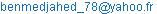(M. Benmedjahed)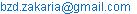(Z. Bouzid)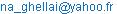(N. Ghellai)

Miloud Benmedjahed, Zakaria Bouzid, Nassera Ghellai. Wind Potential Assessment of Ain Salah in Algeria; Calculation of the Cost Energy. International Journal of Energy and Power Engineering. Vol. 4, No. 2, 2015, pp. 38-42

Abstract: The objective of this work is to evaluate the wind resource on the site of Ain Salah, in Algeria. The hourly data used in this study span a period of 10 years. The parameters considered are the speed and direction of wind. The wind energy production was evaluated and an economic evaluation was conducted for wind farm (10MW) consisting of Twelve WGT 850 KW. The obtained results for the Weibull parameters was k = 2.48 and C = 6.0 m/s, at 10 m above the ground with an average wind speed of 5.4 m/s and the price per kW for our wind frame are 0.1603 USD/Kw.

Keywords: Wind, Wind Rose, Weibull, Energy, Cost, Algeria

1. Introduction

In Algeria, the objectives established by the join-stock company NEAL (New Energy Algeria), focused on raising renewable energy production to 1400 MW in 2030 and 7500 MW at the beginning of 2050. Electrical power will be obtained from solar power plants, which are exclusively solar, or from hybrid solar plants, which also use other forms of renewable or conventional energy, preferably natural gas .

Harnessing the wind is one of the cleanest, most sustainable ways to generate electricity. Wind power produces no toxic emissions and none of the heat-trapping emissions that contribute to global warming. This, and the fact that wind power is one of the most abundant and increasingly cost-competitive energy resources, makes it a viable alternative to the fossil fuels that harm our health and threaten the environment. Wind energy is the fastest growing source of electricity in the world.

In 2012, nearly 45000 MW of new capacity were installed worldwide. This stands as a 10 percent increase in annual additions compared with 2011 .

Many work indicated that Algeria was characterized by a competitive electricity generation cost per kW from Wind turbine, in particular, we can cite the evaluation of electricity generation and energy cost of wind energy conversion systems in southern Algeria  and Assessment of wind energy and energy cost in Algeria .

In this study, we propose to do an analysis of the wind resource and an estimation cost of a wind farm in Ain Salah. This farm consists of twelve wind turbines WGT 850 KW. We will take into account in the calculations the wind characteristics, the geographical position and the varying of air density depending on the temperature.

2. Site & Weather Data

In this study, the wind speed data were collected over a period of 10 years. The details of the site are summarized in table 1.

Table 1. Geographical coordinates of the data collection stations used in the study

 Location Latitude Longitude Altitude Ain Salah 27.25 ° N 2.51°E 269 m

The meteorological measurements were made at 10 meters above ground level and registered every 3 hours. The geographical location of the metrological station is shown in figur1.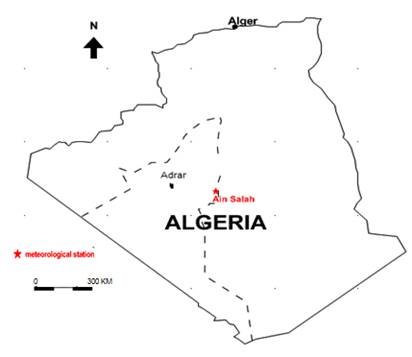Figure 1. Distribution of meteorological stations over Algeria.

3. Methodology

3.1. Weibull Distribution

The wind characteristics will determine the amount of energy that can be effectively extracted from the wind farm. In order to determine the properties of a site, measurements of the speed of wind and its direction are needed.

However, previous studies in the field of wind energy showed that the most important and appropriate characteristic to exploit is the Weibull statistical distribution. Its density probability function can be expressed as :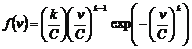(1)

k and C are the shape parameter (dimensionless) and the scale parameter (m/s), respectively. Usually, the shape parameter characterizes the symmetry of the distribution. The scale parameter is very close to the average speed of wind. The standard deviation method was chosen to determine both factors k and C. This method is based on the calculation of the standard deviation and the average speed .

If the wind distribution is desired at some height other than the measurement height, the Weibull parameters can be adjusted to any desired height by the model of Justus .

3.2. The Capacity Factor and Wind Energy

The power contained in an air mass that flows at a speed v through a circular section s can be expressed by the following relationship :(2)

Where ρ is density of air (kg/m³).

A wind turbine allows extracting the kinetic energy from the wind and converting it into mechanical and electric energy. Effective power can be expressed by the following equation :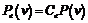(3)Figure 2. Efficiency and power as function of wind speed for WGT 850 KW 

Where Ce is the wind turbine efficiency. The efficiency of the wind turbines taken into consideration in this study are shown in figure 2 and technical specifications of the selected wind turbines are listed in Table 2.

Table 2. Technical specifications of the considered wind turbine 

 Characteristics WGT 850KW Rated power 850KW Rotor diameter 52 m Hub height 55 m Cut-in wind speed 4  m/s Rated wind speed 16 m/s Cut-out wind speed 28 m/s

The capacity factor is defined as the ratio of the average power output to the rated output power of the wind turbine. It can be calculated as follows :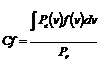(4)

The annual energy generated by a wind turbine can be calculated as follows: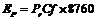(5)

3.3. Energy Cost

The initial investment cost is equal to the sum of costs of components. The total investment cost is given as below :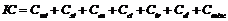(6)

where Cwt is cost of the wind turbine, Cst is Study cost, Cen is engineering cost, Cci is civil work and installation cost,  Ctr  is cost of transport ,Cel is Cost of electrical connection,  Cmisc is miscellaneous cost .The cost break-up of a typical wind frame project are shown in figure 3.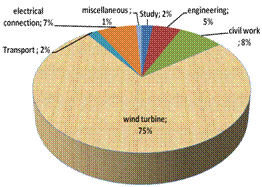Figure 3. Cost break-up of a typical wind frame project 

Cost of wind turbines can vary significantly depending on the type of stock availability and cost of raw materials (steel). The variation can be up to 25-30% of the price. This difference is not negligible because the total cost of purchasing wind turbines is generally 74% of the total project cost. The cost of wind turbine can be determined as follows :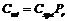(7)

Where Pr is the rated power and Cspe is the specific cost of turbine systems. It is summarized in table3;

Table 3. Specific cost of wind 

 Wind turbine size (kW) Specific  cost (USD/kW) 10–20 2200–2900 20–200 1500–2300 200> 1000–1600

The present value of costs (PVC) can be calculated as follows :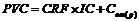(8)

Where: Com (p) is the maintenance costs during the system life (USD). It can be calculated as follows :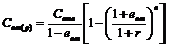(9)

Where Com is the operation and maintenance cost for the ﬁrst year, eoma is escalation of the operation and maintenance cost.

CRF is the capital recovery factor for wind turbine, it can be calculated as follows [8,11]: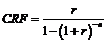(10)

The unite cost energy is the ratio of present value of costs PVC of project on produced electricity :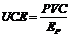(11)

3.4. Planning of the Wind Farm

To produce a large amount of energy, a wind farm must be installed as followed. We use WGT 850 kW wind turbine model.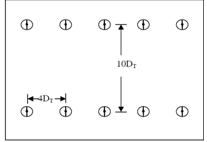Figure 4. Planning of a wind farm 

When several turbines are installed in block, the turbulence due to rotation of the turbine blades can affect other turbines nearby. To minimize this effect, the spacing of about 3 to 4 DT (with DT the diameter of the rotor) is provided inside the rows .

Similarly, the spacing between the rows may be of the order of 10DT, so that the air stream passing through a turbine is restored before its interaction with the following turbine. This spacing can be further increased for better performance but this implies more land used. In general, the energy loss due to the effect of park is about 5%.

4. Results & Discussion

4.1. Wind Data Resource

The present study is based on data source measured at a height of 10 m above ground level, for Ain Salah in Algeria. The wind data were recorded every three hour during ten years (from 2003 to 2013), and were obtained from the Algerian meteorological national office

The shape parameter value for Ain Salah, k = 2.48, shows that distribution is stable and the analysis of the scale parameter, C = 6 m/s, shows that it windy. The Annual mean wind speed and Weibull parameters at 10 m from the ground level of the site are summarized in table 4.

Table 4. Annual mean wind speed and Weibull parameters at 10 m from the ground level

 Location C (m/s) k v (m/s) Ain Salah 6.0 2.48 5.4

Statistical data analysis allowed the determination of wind rose for ten years. The results obtained (see figure 5) show that:

In Ain Salah, the East-North-East (ENE) sector represents 30 % of wind frequencies.

The eastern sector are predominant sectors with a percentage of around 14 %.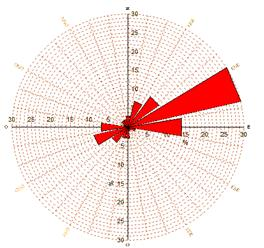Figure 5. The wind rose for studied site at 10m height.

4.2. Power and Produced Energy

The value of annual average power, capacity factor and energy produced for WGT 850 are summarized in table5

Table 5. Average power, annual capacity factor and energy production in Ain Salah

 WGT Wind frame Power (kW) 204.12 2327.02 Capacity factor (%) 24. 01 23.27 Energy (GWh) 1.79 20.38

4.3. Cost Energy

The estimation of the costs of the kWh of energy delivered by the WGT 850, at the considered site has been done under the following assumptions :

The lifetime of the WGT (n) was assumed to be 20 years.

The discount rate (r) was taken as 8%.

The escalation ratio of operation and maintenance (em) was assumed to 6%.

The operation and maintenance cost for the ﬁrst year (Com) was considered to be 25%.

It is assumed that the WGT produces equal amount of energy output in each year during its useful lifetime.

The average values of the costs of the study, engineering, civil work, wind turbines, transport, electrical connection, miscellaneous components and other costs are summarized in table 6.

The cost per kWh of energy produced depends on the wind turbine type as well as the wind characteristics of the site. The results of cost per kWh for our wind frame are 0.1603 USD/kWh.

Table 6. The wind frame cost

 Costs Values Study 265200 USD Engineering 663000 USD Civil work 1060800 USD Wind turbine 9945000 USD Transport 265200 USD Electrical connection 928200 USD Miscellaneous 132600 USD Initial Investment cost 13260000 USD Maintenance Cost 1466700 USD Present value cost 3267400 USD Unite cost energy 0.1603 USD/KW

4.4. Wind Farm Planning

In order to reach the nominal power 10 MW of the wind farm, our choice is focused on one row of twelve wind turbines from South-South-East (SSE) to North-North-West (NNW), 208 m of distance between each two turbines. Geographical wind farm planning is illustrated in figure 6 and the virtual wind farm is shown in figure 7.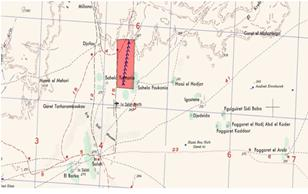Figure 6. Geographical wind farm planning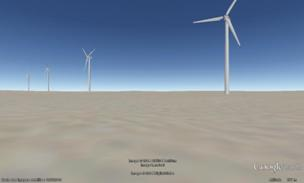Figure 7. the virtual wind farm

For visual impact, we can say that if the wind farm was constructed cleverly, they can be a positive addition to the landscape.

5. Conclusion

This study focused on the evaluation of wind potential and the cost per KW of energy produced in Ain Salah, based on wind speed measurements recorded during a ten-year period, from 2003 to 2013. Wind resource analysis in the selected site shows that south Algeria has a wind energy potential that can be exploited effectively. Indeed, statistical treatment of data allowed evaluating the characteristic speeds and wind potential. The results obtained show that:

The shape parameter value k for Ain Salah equal to 2.48. It shows that distribution is stable and the analysis of the scale parameter C shows that it windy (6m/s).

The East-North-East (ENE) sector represents 30 % of wind frequencies, while the eastern sector is predominant sectors with a percentage of around 14 %.

The value of annual average power, capacity factor and energy produced for WGT 850 KW account respectively 204.12 kW, 24% and 1.79 GWh.

The total annual average power, capacity factor and energy produced for wind frame account respectively 2327.02 kW, 23.27% and 20.38 GWh.

The average values of the costs of the study, engineering, civil work, wind turbines, transport, electrical connection and miscellaneous components are 265200 USD, 663000 USD, 1060800 USD, 9945000 USD, 265200 USD, 928200 USD and 132600 USD respectively, which the initial investment cost  represents 13260000 USD.

The average values of the maintenance cost and present value cost are 1466700 USD and 3267400 USD respectively.

The average values of the maintenance cost and present value cost are 1466700 USD and 3267400 USD respectively.

The cost per KW for our wind frame are 0.1603 USD/KW

If the wind farm was constructed cleverly; they can be a positive addition to the landscape.

References

1. T. Hasni, " Développement des Energies Renouvelables en Algérie".In National Conference on Renewable Energies and Sustainable Development. Laghouat, Algeria,2006.
2. A .Mirecki, "Etude comparative de chaînes de conversion d’énergie dédiées à une éolienne de petite puissance" , docteur de l’institut national polytechnique de Toulouse,France,  2005.
3. S.Daif, M.Belhamel, M.Haddadi, A.Louche,"Technical and economic assessment of hybrid photovoltaic/wind system with battery storage in Corsica island". Energy policy, 2008,36:743-754.
4. M.Benmedjahed , N.Ghellai, A.Benmansour ,SM.Boudai  , M A .Tabet Hellal , "Assessment of wind energy and energy cost in Algeria",International Journal of Renewable Energy,2014 ,Vol. 9, No: 1,32-39
5. G .BoroumanJazi, B .Rismanchi, R.Saidur,"Technical characteristic analysis of wind energy conversion systems for sustainable development".Energy Convers Manage,2013 ,69:87–94.
6. M.Sathyajith,"Wind energy fundamentals, resource analysis and economics". Berlin (Heidelberg, Netherlands): Springer-Verlag,2006.
7. C.Justus, WR.Hargraves, A.Mikhaeil,D.Graber,"Methods for estimating wind speed frequency distributions",J Appl Meteorol , 1977.17:350–353.
8. A.Arbaoui , "Aide a la décision pour la définition d’un système éolien adéquation au site et a un réseau faible.  Thèses doctorat", École Nationale Supérieure d’Arts et Métiers Centre de Bordeaux,France, 2006.
9. EMD, 2012.The wind pro software. WindPRO Data\WTG Data, version 2.8.543.
10. M. Duquesne, "Eolien: quelle est la répartition des coûts entre les différents postes d’un projet Rapport ", Union des Villes et Communes de Wallonie asb.2010.
11. M.Gökçek, Genç M S."Evaluation of electricity generation and energy cost of wind energy conversion systems (WECSs) in Central Turkey". Appl Energy,2010,86:2731–2739.

 Contents 1. 2. 3. 3.1. 3.2. 3.3. 3.4. 4. 4.1. 4.2. 4.3. 4.4. 5.
Article ToolsAbstractPDF(814K)